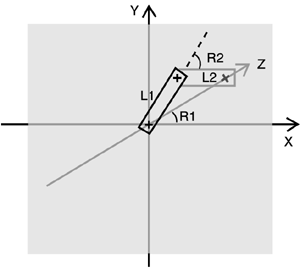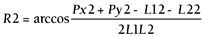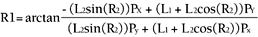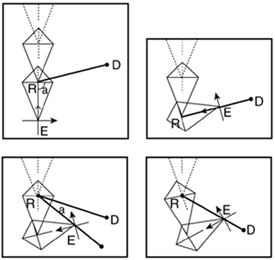# Inverse Kinematics

IK works from the terminal nodes and solves the transforms for the whole hierarchy, so the terminal has a certain position and orientation. It is used widely in movies, and it is slowly finding its way into games. Grabbing objects, keeping feet on the ground realistically, and character-to-character interaction are just some of the uses of IK.

From a programming standpoint, IK starts with some restrictions (usually, a terminal location and orientation) and tries to find solutions depending on the skeleton geometry. To find such solutions, some approaches use analytic methods, computing solutions from problem-specific equations. These methods, when possible to use, are the fastest at the cost of lower generality. Then, for complex configurations, iterative processes are used, much like in numerical analysis where an exact solution is found by progressive refinement, such as in the popular hill-climbing, problem-solving method. We will now take a look at two examples, one using an analytic solver and another using Cyclic Coordinate Descent (CCD), which is one of the most popular iterative algorithms.

#### Analytic IK

Analytic IK solutions are found by describing the animation system we want to model in terms of equations and solving them. For simple systems, this can be computed in real time and add a greater sense of control to the player. For example, a custom analytic solver could be designed to animate the shoulder-elbow-wrist system in an RPG, so we can make the character grab objects or open doors realistically. If we know the constraints beforehand, and the number of joints is small, analytic IK is the way to go.

Take a single bone that can rotate around a single axis, for example. Given a rotation angle R1 and a bone length L1, the endpoint of the bone is

` Px = L1*cos(R1) Py = L1*sin(R1) Pz = 0 `

Notice I assumed that the bone rotates in the X,Y plane and is centered at the origin. Now, imagine that we concatenate that bone to a second one in a chain.

The second bone is expressed in terms of length L2 and rotation R2 (see Figure 15.8). The equation for the endpoint of the second bone in terms of L1 L2 and R1 R2 is

` Px = L1*cos(R1) + L2*cos(R1+R2) Py = L1*sin(R1) + L2*sin(R1+R2) `
##### Figure 15.8. Two-bone chain as used for analytic IK.This gives us positions based on bone configurations, which is what we have been calling FK so far. It is now time to inverse these equations and represent R1 and R2 (the real parameters, because L1 and L2 can be considered constants) in terms of a predefined Px,Py pair. Thus, the equation must give us the joint orientations required to reach one endpoint in particular. Now, we will need some algebra to get this working. To begin with, we will use the following identities:

` cos(a+b) = cos(a)*cos(b)   sin(a)*sin(b) sin(a+b) = cos(a)*sin(b) + sin(a)*cos(b) `

Coupling these with our FK equations and squaring both FK equations and then adding them, we reach the following:

` PX2 + PX2 = L12 + L22 + 2L1L2cos(R2) `

which can be trivially solved into:And substituting we get:This is the analytical solution to our IK problem, assuming it's happening in 2D. Similar approaches can be used for 3D, or we can simply rotate the bones so they lie in one of the coordinate planes.

Now, you need to notice several relevant ideas. First, notice how the equations introduce the concept of reachability. Take a look at the way we compute R2. It's an arc-cosine. Thus, it is assuming the argument is in the range between 1 and 1. So what happens if this isn't so? Obviously, the equations would have no solution, and the geometric interpretation would be that the point Px,Py is just too far.

Second, notice how reachability does not imply the use of restrictions. Imagine that this two-link system is indeed the upper and lower parts of an arm. Maybe the proposed solution will imply bending it in ways we humans simply cannot do. Pure IK does not handle that. We would need additional equations to take care of restrictions. In the case of a human elbow (which would be controlled by R2), we would add:

` R2>0 R2<Pi `

to represent the fact that an elbow can actually only bend approximately 180 degrees.

The third interesting fact about all this is that sometimes more than one solution will appear. In our case, I guess it's fairly clear that two solutions are always possible, as shown in Figure 15.9.

##### Figure 15.9. Different solutions are often frequent in inverse kinematics.Analytic solutions are used frequently in games, because they are easy to solve and can handle many game-specific problems. Need a character to reach out with a hand? The preceding code is perfectly well suited for this, because the arm-hand is not very relevant, and we need to focus on the shoulder and elbow. Need a character to place his feet on the ground? You can adapt the preceding equations easily, maybe adding a third restriction that represents the angle at which the foot must stand on the ground, probably derived from the slope. But what about general IK solvers? There are numerical methods available that can solve more or less anything by using iterative processes. By using them, more complex systems with many restrictions can be analyzed. One of the most popular algorithms is the CCD. Let's take a look at it.

#### Cyclic Coordinate Descent

CCD (introduced in 1993 by Chris Welman) works by analyzing joints one by one in a progressive refinement philosophy. It starts with the last joint in the chain (the hand, for example) and tries to rotate it to orientate it toward the target. Then, it moves one link up, and so on. Let's take a look at the example in Figure 15.10.

##### Figure 15.10. CCD.We start at the last link in the chain. If the target was effectively within reach, that would mean we would need to rotate that bone to aim at the goal. Thus, we compute that hypothetical rotation. This is easy, especially if you've read the chapter on action AI. It is like a starship rotating to face an enemy. We create two vectors: one from the last joint position to the endpoint of the last bone (the effector) and another from the same last joint to the target we are trying to reach. Now we can compute the angle between these two vectors by computing the dot product of their unit vectors. Notice that we have the angle between two vectors, but we need to make sure we rotate in the appropriate direction to make the shortest path possible. Again, we can use our AI knowledge by performing a cross product between the two vectors to obtain a third, perpendicular vector. Then, we use the sign of the Z coordinate of the newly computed vector. This sign determines the direction at which we must rotate, and the arc-cosine of the dot product will give you the angle.

Now we can move up one link and repeat the angle rotation process. In the end, this is a progressive refinement algorithm that will eventually leave the last bone's endpoint close enough to the target, or bail out. But remember that this is just a computational algorithm. The results are not meant to be shown to the user because the process of reaching out to the target by CCD does not necessarily look very human. What we would actually do is use the angles computed as results of the CCD process to drive a quaternion or matrix interpolator, which would create the animation. Thus, CCD is a preprocess we perform the moment we decide we must reach out for something.

Here is the pseudocode for the CCD system I have just outlined. I'll assume we are starting at the final bone in the system.

` While distance from effector to target > threshold and numloops<max       Take current bone       Build vector V1 from bone pivot to effector       Build vector V2 from bone pivot to target       Get the angle between V1 and V2       Get the rotation direction       Apply a differential rotation to the current bone       If it is the base node then the new current bone is the last bone in the chain       Else the new current bone is the previous one in the chain End while `Core Techniques and Algorithms in Game Programming2003
ISBN: N/A
EAN: N/A
Year: 2004
Pages: 261